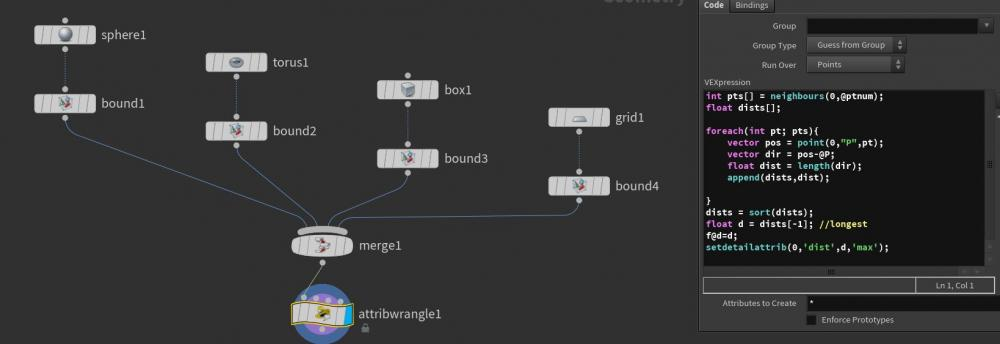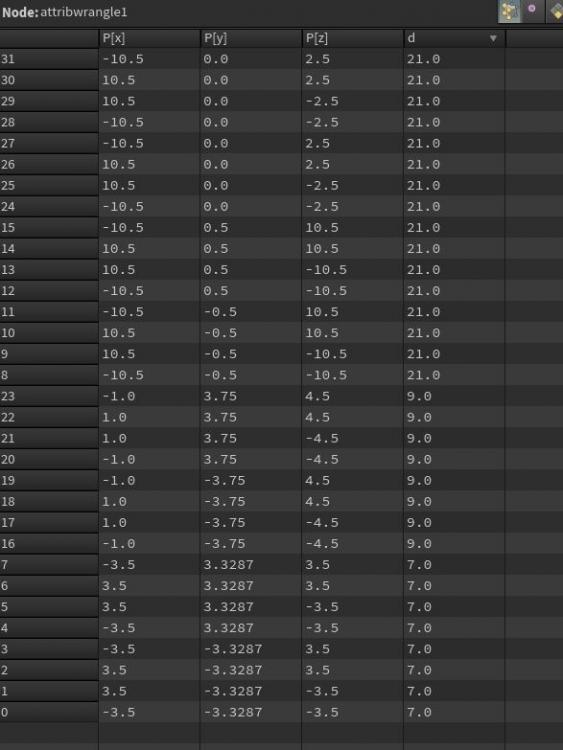# VEX: find the max of the max of bounding boxes of connected pieces

## Recommended Posts

Posted (edited)

For each connected piece, get the bounding box, find the axis with the maximum scale and remember just the number. Say e.g. a box is 18 by 3 by 2, a grid is 4 by 7, a sphere is 15 by 15 by 15 then we need 18, 15, and 7.

Then find the max of these maxs (18 vs 7 vs 15) and assign it to an attribute, such that we end up f@maxattrib = 18.

Edited by anicg

##### Share on other sites

Put to point wrangle

```
int pts[] = neighbours(0,@ptnum);
float dists[];

foreach(int pt; pts){
vector pos = point(0,"P",pt);
vector dir = pos-@P;
float dist = length(dir);
append(dists,dist);

}
dists = sort(dists);
float d = dists[-1]; //longest
f@d=d;
setdetailattrib(0,'dist',d,'max');```

in first input connect all bounding boxes

##### Share on other sites
On 25/06/2020 at 8:26 PM, tamagochy said:

Put to point wrangle

```

int pts[] = neighbours(0,@ptnum);
float dists[];

foreach(int pt; pts){
vector pos = point(0,"P",pt);
vector dir = pos-@P;
float dist = length(dir);
append(dists,dist);

}
dists = sort(dists);
float d = dists[-1]; //longest
f@d=d;
setdetailattrib(0,'dist',d,'max');```

in first input connect all bounding boxes

Thank you, I'm almost there, I get an attribute d which has 7,9 and 21, I only need the 21, the highest number, one value, what do I need to add to get that?##### Share on other sites

you can also just directly merge your geo (without appending Bound SOP to each)

use Connectivity in Point mode to compute connectivity attribute, default i@class for example

then Point Wrangle:

```vector scale = getpointbbox_size(0, "@class=" + itoa(i@class));
float max = max(scale);
setdetailattrib(0, "max", max, "max");```

should give you what you need

##### Share on other sites
On 7/8/2020 at 6:51 PM, anim said:

you can also just directly merge your geo (without appending Bound SOP to each)

use Connectivity in Point mode to compute connectivity attribute, default i@class for example

then Point Wrangle:

```
vector scale = getpointbbox_size(0, "@class=" + itoa(i@class));
float max = max(scale);
setdetailattrib(0, "max", max, "max");```

should give you what you need

Is the getpointbbox working in world coordinates? But if you need oriented bounds this code not enough I thinks.

##### Share on other sites

Right, it doesn't do oriented bounding box

You would need to transform your pieces by inverted oriented transform before that node to get the oriented bbox lengths

Unless they are packed and then oriented in which case you can use bounds intrinsic

## Create an account

Register a new account